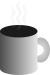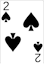#Coffee SpaceListen:

## End of the Line

I think the Universe will come to an end one way or another, after all “Everything that has a beginning has an end, Neo”. More substantial than that would be that with the assumption the Universe is a closed system and the physics of that system can be reduced to a Langton’s Ant simulation, there are a finite number of states the Universe can occupy and therefore it will eventually return back to it’s original state even if that means expressing every individual state.

Of course, it’s not needed that every state is explored as rotation in a 3D space reduces this (if the universe expanded uniformly). I suppose this also reduces the Universe to an incredible number of states, but a number of states that could be calculated (at least in theory).

### Space vs Time vs Matter vs Energy

I propose these are all the same thing. Time is a bi-product of space - the relative speed difference in which space can pass space as I think Space = Mass = Energy. I think that for a stationary object, the following applies:

```0001 E = m.c^2 = k.s
0002   Where E=Energy, m=mass, c=constant(speed of light),
0003   k=constant(unknown) and s=space```

Time is a measurement/result of space so doesn’t get consideration here. The only thing I am to figure in this model is electro fields. It doesn’t yet fit the model.

### Dark Energy

If this is right I think the need for dark energy disappears. Dark energy has been predicted to be everywhere, pulling things together all around us. I think the reason we can’t find it is because it isn’t there and it’s something we automatically dismiss - space! Imagine that only large amounts of space have measurable gravity. Singly these “clumps” don’t amount to anything measurable but together they represent vast amounts of gravity. Gravity is the stretching of space and vast amounts of stretched space must hence have gravity. It makes perfect sense.